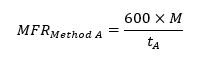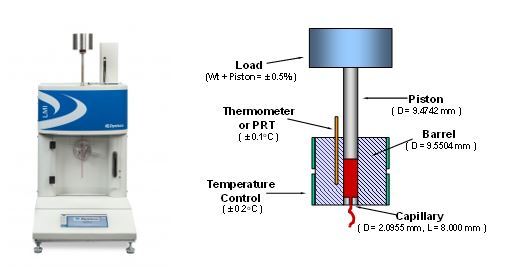# Methods for Measuring the Melt Flow Rate of Thermoplastic Materials

A flow rate test is used to work out the melt flow rate (MFR) of a thermoplastic material. According to ASTM 1238, “Method A” test involves the collection of extrudate from the die over a set period of time, under specific conditions of load and temperature. Mass melt flow rate can then be calculated based on the mass of the extrudate(s) using the equation below:where is the mass in grams of material collected over time. The MFR value is measured in units g/10 min (grams extruded in 10 minutes). “Procedure A” is completely manual and is sometimes known as the “cut-n-weight” method.

Method B test is an assessment of the flow characteristics of a material based on the volumetric displacement, rather than weight of extrudate with time, as in Method A.Image Credit: Dynisco

The extrudate does not need to be cut and weighed in Method B, unlike in Method A. Results from Method B can be expressed directly as Melt Volume-flow Rate (MVR) in cc/10 min. In order to relate the results from Method B back to Method A, the apparent melt density calculated from Method A to B conversion is required. Since Method B is a “no-cuts” test, it is more precise and simpler to run for routine analysis.

The MVR from Method B can be calculated based on the volumetric displacement of the extrudate(s) using the equation below:Where R is barrel rations (R = 0.477 cm) and tb is the time of the piston travel for distance L (flag length). Based on ASTM 1238, L = 0.635 cm. The value of “600” is used in order to express the unit of MVR as cc/10 min.

The value of the MFR (g/10 min) is given by multiplying the calculated MVR by melt density of the material, as shown by the equation below:

MFRMethod B = MVR x ρm

where ρm (g/cm3) is the apparent melt density of the polymer at the test temperature. It is important to note that the melt density of the polymer is also the function temperature. Reinforcing agents, fillers, etc. tend to increase the melt density of the material.

This test method is useful for quality control materials from different processes or supplier lots. MFR is a parameter that is empirically defined and influenced by the molecular structure and physical properties of the polymer material, such as molecular weight, viscosity, moisture in material, molecular weight distribution, crosslinking, additives, or degradation. This test can also be used to determine some polymer characteristics, for example extrudate swell, shear thinning of the polymer melt, intrinsic viscosity and thermal stability.

## Acknowledgments

Produced from materials originally authored by Azadeh Farahanchi, Rheological Scientist, Ph.D.This information has been sourced, reviewed and adapted from materials provided by Dynisco.

## Citations

• APA

Dynisco. (2020, April 27). Methods for Measuring the Melt Flow Rate of Thermoplastic Materials. AZoM. Retrieved on October 19, 2020 from https://www.azom.com/article.aspx?ArticleID=19173.

• MLA

Dynisco. "Methods for Measuring the Melt Flow Rate of Thermoplastic Materials". AZoM. 19 October 2020. <https://www.azom.com/article.aspx?ArticleID=19173>.

• Chicago

Dynisco. "Methods for Measuring the Melt Flow Rate of Thermoplastic Materials". AZoM. https://www.azom.com/article.aspx?ArticleID=19173. (accessed October 19, 2020).

• Harvard

Dynisco. 2020. Methods for Measuring the Melt Flow Rate of Thermoplastic Materials. AZoM, viewed 19 October 2020, https://www.azom.com/article.aspx?ArticleID=19173.

## Tell Us What You Think